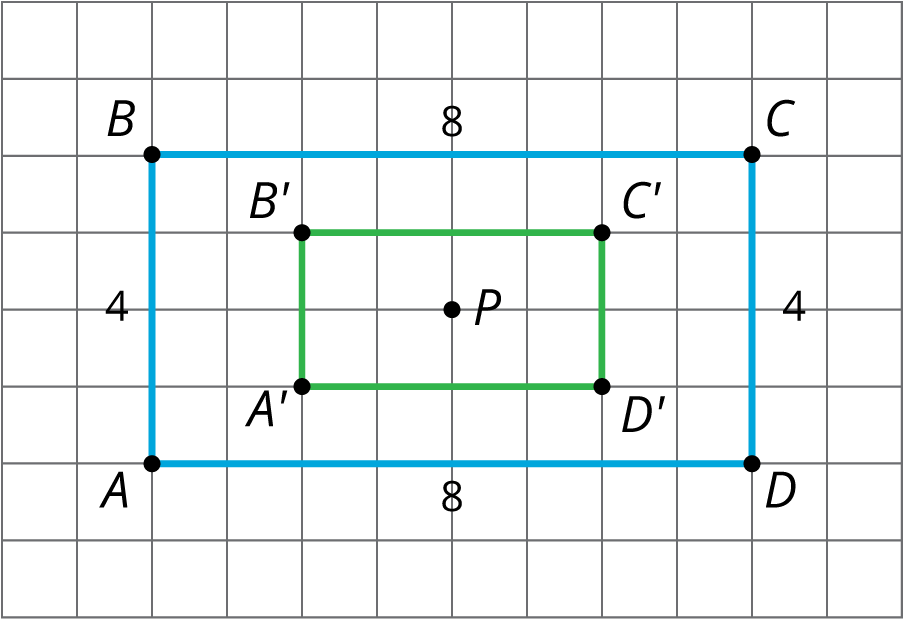## Dilations

This week your student will expand their understanding of transformations to include non-rigid transformations. Specifically, they will learn to make and describe dilations of figures. A dilation is a process to make a scaled copy of a figure, and is described using a center point and a number (the scale factor). The scale factor can be any positive number, including fractions and decimals. If the scale factor is less than 1, the dilated figure is smaller than the original, if it is greater than 1 the dilated figure is larger than the original. In this dilation, the center point $P$ and the scale factor is $\frac12$.When dilating figures, the distance from the center of dilation to a point on the figure is multiplied by the scale factor to get the location of the corresponding point. In this example, the distance between center $P$ and $B$ multiplied by $\frac12$ results in the distance between $P$ and $B'$. Notice also how the side lengths of the dilated figure, $A’B’C’D’$ are all exactly $\frac12$ the side lengths of the original figure, $ABCD$, while the angle measures remain the same.

Here is a task to try with your student:

Rectangle A measures 10 cm by 24 cm. Rectangle B is a scaled copy of Rectangle A.

1. If the scale factor is $\frac12$, what are the dimensions of Rectangle B?
2. If the scale factor is 3, what are the dimensions of Rectangle B?
3. If Rectangle B has dimensions 15 cm by 36 cm, what is the scale factor?

Solution:

1. Rectangle B has dimensions 5 cm by 12 cm, since $10 \boldcdot \frac12=5$ and $24 \boldcdot \frac12=12$.
2. Rectangle B has dimensions 30 cm by 72 cm, since $10 \boldcdot 3=30$ and $24 \boldcdot 3=72$.
3. The scale factor is $\frac32$ since $15 \div 10=\frac32$ and $36 \div 24=\frac32$.

## Similarity

This week your student will investigate what it means for two figures to be similar. Similarity in mathematics means there is a sequence of translations, rotations, reflections, and dilations that takes one figure to the other. When two figures are similar, there are always many different sequences of transformations that can show that they are similar. Here is an example of two similar figures:If we needed to show that these two figures are similar, we can first identify that the scale factor to go from $ABDC$ to $EFHG$ is $\frac34,$ since $3 \div 4 = 4.5 \div 6 = \frac34.$ Then, using a dilation with scale factor $\frac34$, a translation, and a rotation, we can line up an image of $ABDC$ perfectly on top of $EFHG.$

Here is a task to try with your student:

Quadrilateral $ABCD$ is similar to quadrilateral $GHEF$.What is the perimeter of quadrilateral $EFGH$?

Solution:

The perimeter is 42. The scale factor is 1.5, since $9 \div 6=1.5$. This means the side lengths of $EFGH$ are 9, 10.5, 7.5, and 15, which are the values of the corresponding sides of $ABCD$ multiplied by 1.5. We could also just multiply the perimeter of $ABCD$, 28, by 1.5.

## Slope

This week your student will use what they have learned about similar triangles to define the slope of a line. A slope triangle for a line is a triangle whose longest side lies on the line and whose other two sides are vertical and horizontal. Here are two slope triangles for the line $\ell$:For lines, it turns out that the quotient of the vertical side length and the horizontal side length of a slope triangle does not depend on the triangle. That is, all slope triangles for a line have the same quotient between their vertical and horizontal side and this number is called the slope of the line. The slope of line $\ell$ shown here can be written as $\frac68$ (from the larger triangle), $\frac34$ (from the smaller triangle), 0.75, or any other equivalent value.

By combining what they know about the slope of a line and similar triangles, students will begin writing equations of lines—a skill they will continue to use and refine throughout the rest of the year.

Here is a task to try with your student:

Here is a line with a slope triangle already drawn in.1. What is the slope of the line?
2. Draw another line with a slope of $\frac43$ that goes through the point on the left. Include a slope triangle for the new line to show how you know this line has a slope of $\frac43$.

Solution:

1. The slope of the line is $\frac37$.
2.